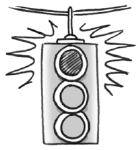### Home > GC > Chapter 12 > Lesson 12.2.3 > Problem12-79

12-79.Jamila solved the quadratic $x^2+3x-10=8$ (see her work below). When she checked her solutions, they did not make the equation true. However, Jamila cannot find her mistake. Explain her error and then solve the quadratic correctly.

$\begin{array}{c} x^2 + 3x - 10 = 8 \\ \left(x+5\right) \left(x-2\right) = 8 \\ x + 5 = 8 \quad \text{or} \quad x - 2 = 8 \\ \; \, x = 3 \quad \text{or} \quad x = 10 \end{array}$

When solving a quadratic equation, it is important to set one side equal to zero.

$x^2+3x-18=0$

$(x+6)(x-3)=0$